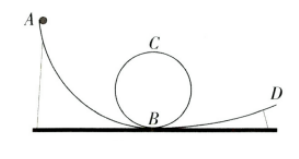$\text{A.}$ 小球从A到B的过程中，机械能增大 $\text{B.}$ 小球从B到C的过程中，重力势能增大 $\text{C.}$ 小球经过C处时速度可能恰好为零 $\text{D.}$ 小球从C到D的过程中，动能转化为重力势能

B

#### 解析：

【解题思路】不考虑一切摩擦阻力, 则小球运动过程中机械能总量不变, $\mathrm{A}$ 错误; 小球从 $\mathrm{B}$ 到 $\mathrm{C}$ 的过程中, 高度增加, 质量不变, 因此重力势能增大, $\mathrm{B}$ 正确; $\mathrm{C}$ 处的高度比 $\mathrm{A}$ 处低, 小球在 $\mathrm{C}$ 处时的重力势能比在 $\mathrm{A}$ 处时 小, 则小球在 $\mathrm{C}$ 处时的动能比在 $\mathrm{A}$ 处时大, 故小球经过 $\mathrm{C}$ 处时动能不为䨐, 速度也不为䨐, $C$ 错误; 小球从C到 $\mathrm{D}$ 的过程中, 小球先降低后升高, 因此先是重力势能转化为动能, 然后一部分动能又转化为重力势能, $\mathrm{D}$ 错 误.